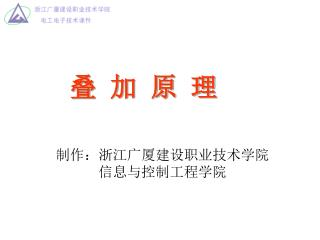# 叠 加 原 理 - PowerPoint PPT PresentationDownload PresentationDownload Presentation## 叠 加 原 理

- - - - - - - - - - - - - - - - - - - - - - - - - - - E N D - - - - - - - - - - - - - - - - - - - - - - - - - - -
##### Presentation Transcript

1. 叠 加 原 理 制作：浙江广厦建设职业技术学院 信息与控制工程学院

2. 叠加原理 1、内容：在多个电源同时作用的线性电路中，任一支路的电流或任意两点间的电压，等于各个电源单独作用时所得电流或电压的代数和。 ★几点说明： （1）线性电路：电路参数不随电压、电流的变化而改变的电路。 （2）电源单独作用：在电路中只保留一个电源，而将其它电源去掉(将电压源的短路、将电流源开路); 电路中所有的电阻连接方式不变。

3. 2、叠加定理应用中的注意事项： （1） 只适用于线性电路。 （2）只将电源分别考虑，电路的结构和参数不变。 暂时不予考虑的恒压源应予以短路，即令E=0； 暂时不予考虑的恒流源应予以开路，即令Is=0。 （3）各电源单独作用时对应支路标明的电流、电压的 方向应与原电路中各电压、电流标明的参考方向 一致。 （4）叠加定理只能用于电压或电流的叠加，不能用来 求功率。

4. 例1：用叠加原理计算图1中的I、URL,已知R1=R2= RL= 1KΩ E1 =9V, E2 =6V。 解：（1）E1单独作用，E2短路，电路如（a）图 I′= E1/（R1+R2+ RL）=3mA （2）E2单独作用，E1短路，电路如（b）图 I″= E2/（R1+R2+ RL）= 2mA （3）叠加： I= I′- I″=3-2=1mA URL=I RL =1V 图1 图（a） 图（b）

5. 例2：电路如图2所示，已知E1=27V，E2=13.5V，R1= 1Ω，R2=3Ω， RL=6Ω，用叠加原理求各支路电流。 解（1）E1单独作用，E2短路，求I1′、I2′、I3′ 电路如图2（a） R总= R1+（R2∥R3）=3Ω I1′ = E1/ R总=27/3=9A I2′ = [R3/（R2+R3）] I1′ =6A I3′ = [R2/（R2+R3）] I1′ =3A 或 I3′= I1′- I2′=3A 图2 图2（a）

6. （2）E2单独作用，E1短路，求I1″、I2″、I3″，电路如图2（b）（2）E2单独作用，E1短路，求I1″、I2″、I3″，电路如图2（b） R总= R2+（R1∥R3）=27/7Ω I2″= E2/ R总=13.5/（27/7）=3.5A I1″ = [R3/（R1+R3）] I2″=3A I3″= [R1/（R1+R3）] I2″ =0.5A 或 I3″ = I2″ - I1″ =0.5A • （3）求原电路图2中各支路的电流I1、I2、I3 I1= I1′- I1″=9-3=6A I2=I2″- I2′=3.5-6= - 2.5A I3= I3′+ I3″=3+0.5=3.5A 图2（b）

7. 例3：已知E=10V，IS=10A，R1= R2=1Ω，试用叠加原理求如图3电路所示 的电流I和电压U 解：此为一个电压源和一个电流源共同作用的电路。电压源单独作用，电流源 应予以开路；电流源单独作用，电压源应予以短路。如果有内阻的话，要 仍留在原处。 （1）电压源单独作用时，电路如图3（a） I′=E/（R1+R2）=10/（1+1）=5A U′= I′R=5*1=5（V） （2）电流源单独作用时，电路如图3（b） I″=[ R1/（R1+R2）]*IS=5A U″= I″R=5*1=5（V） 图3 （3）叠加原理，求I 和 U I=I′+I″=5+5=10A U=U′+U″=5+5=10V 图3（a） 图3（b）

8. 小结： 1、叠加原理，是指在一个包含多个电源的线性电路中，各支路的电流（电压）等于各个电源分别单独作用时，在各支路所产生的电流（电压）的代数和。 2、叠加原理适用于电压和电流的，不适用功率的叠加。 作 业：见参考书（一）第191页 9-17